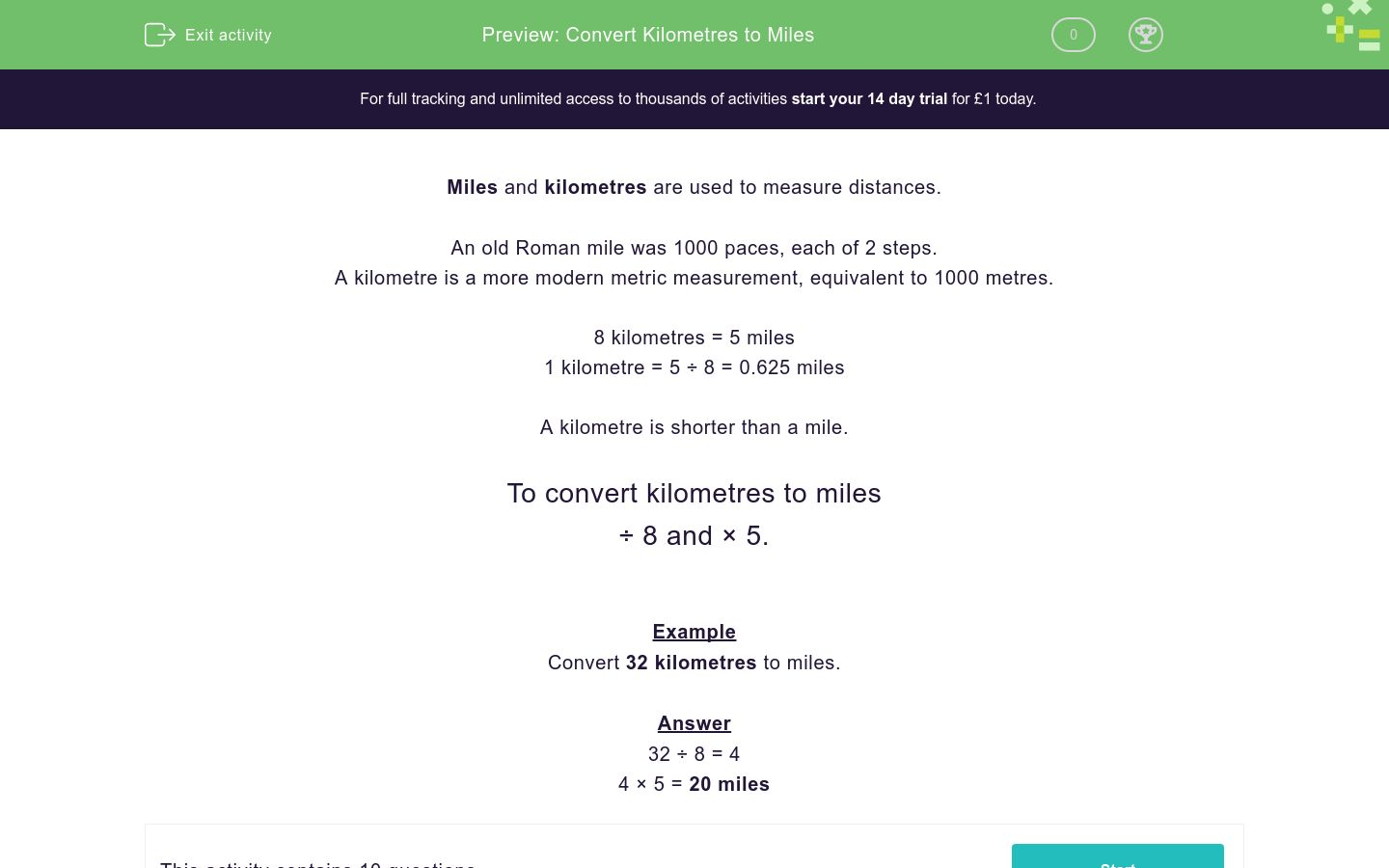# Convert Kilometres to Miles

In this worksheet, students must convert distances from kilometres to miles.Key stage:  KS 2

Curriculum topic:   Measurement

Curriculum subtopic:   Convert Miles/Kilometres

Difficulty level:### QUESTION 1 of 10

Miles and kilometres are used to measure distances.

An old Roman mile was 1000 paces, each of 2 steps.

A kilometre is a more modern metric measurement, equivalent to 1000 metres.

8 kilometres = 5 miles

1 kilometre = 5 ÷ 8 = 0.625 miles

A kilometre is shorter than a mile.

To convert kilometres to miles

÷ 8 and × 5.

Example

Convert 32 kilometres to miles.

32 ÷ 8 = 4

4 × 5 = 20 miles

Convert to miles:

16 kilometres

(Just write the number.)

Convert to miles:

24 kilometres

(Just write the number.)

Convert to miles:

40 kilometres

(Just write the number.)

Convert to miles:

56 kilometres

(Just write the number.)

Convert to miles:

48 kilometres

(Just write the number.)

Convert to miles:

72 kilometres

(Just write the number.)

Convert to miles:

880 kilometres

(Just write the number.)

Convert to miles:

960 kilometres

(Just write the number.)

Which is further?

52 miles

80 km

Which is further?

158 miles

248 km

• Question 1

Convert to miles:

16 kilometres

(Just write the number.)

10
EDDIE SAYS
16 ÷ 8 = 2
2 × 5 = 10 miles
• Question 2

Convert to miles:

24 kilometres

(Just write the number.)

15
EDDIE SAYS
24 ÷ 8 = 3
3 × 5 = 15 miles
• Question 3

Convert to miles:

40 kilometres

(Just write the number.)

25
EDDIE SAYS
40 ÷ 8 = 5
5 × 5 = 25 miles
• Question 4

Convert to miles:

56 kilometres

(Just write the number.)

35
EDDIE SAYS
56 ÷ 8 = 7
7 × 5 = 35 miles
• Question 5

Convert to miles:

48 kilometres

(Just write the number.)

30
EDDIE SAYS
48 ÷ 8 = 6
6 × 5 = 30 miles
• Question 6

Convert to miles:

72 kilometres

(Just write the number.)

45
EDDIE SAYS
72 ÷ 8 = 9
9 × 5 = 45 miles
• Question 7

Convert to miles:

880 kilometres

(Just write the number.)

550
EDDIE SAYS
880 ÷ 8 = 110
110 × 5 = 550 miles
• Question 8

Convert to miles:

960 kilometres

(Just write the number.)

600
EDDIE SAYS
960 ÷ 8 = 120
120 × 5 = 600 miles
• Question 9

Which is further?

52 miles
EDDIE SAYS
80 km = 80 ÷ 8 × 5 = 50 miles
• Question 10

Which is further?

158 miles
EDDIE SAYS
248 km = 248 ÷ 8 = 31 × 5 = 155 miles
---- OR ----

Sign up for a £1 trial so you can track and measure your child's progress on this activity.

### What is EdPlace?

We're your National Curriculum aligned online education content provider helping each child succeed in English, maths and science from year 1 to GCSE. With an EdPlace account you’ll be able to track and measure progress, helping each child achieve their best. We build confidence and attainment by personalising each child’s learning at a level that suits them.

Get started